# Kruscal算法正确性证明

### 贪心算法：

1.每次的选择一定是当前的最佳选择

2.只看眼前的利益，不把眼光放长远，不像回溯，贪心没有后悔药

1.最优子结构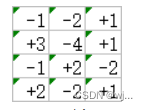2.贪心选择性

### Kruscal算法：

1、每次选择最小的边

2、如果最小的边加入到已选的边以后不会产生回路，则在剩余的边中继续，否则去掉这条边

### 如何证明Kruscal算法的正确性：

e不在U中，说明把e加到U中一定会形成环(因为U是最小生成树，定义)

(其实环路中，除了e，其他边肯定不在T中，如果在T中，T就加不上e,因为会有回路)

### Kruscal算法实现：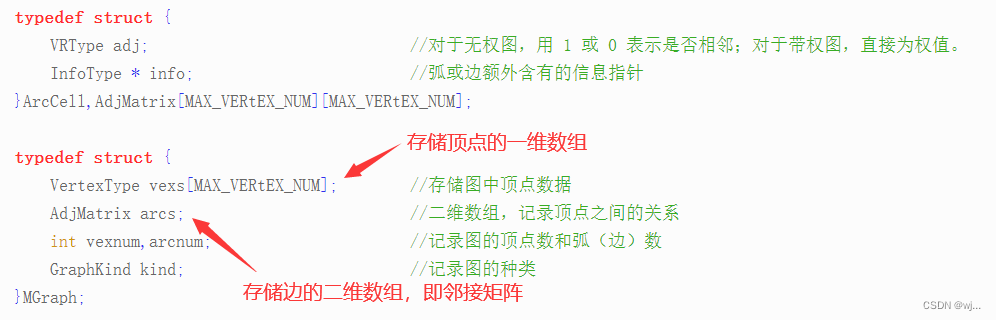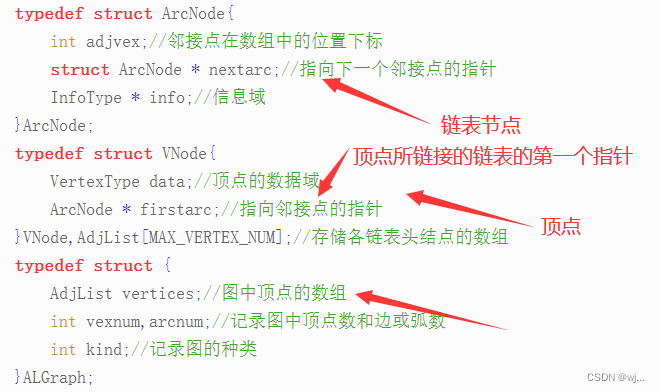package Graph;

import java.util.Scanner;

public class Kruscal {
//最小生成树 用邻接表存储
//构造树时，需要有边的集合和点的集合
public int arcnum;
public int vexnum;
edge[] edges;
vertex[] vertices;
//我们用的属于内部类,static+内部类相当于把它变成了外部类
class edge{
//三个内容，边的起点值，终点值，权值
public int start;
public int end;
public int weight;

public edge() {
}

public edge(int start, int end, int weight) {
this.start = start;
this.end = end;
this.weight = weight;
}
}
//每个顶点也用一个类来表示
class vertex{
//两个内容，边的值以及边所属集合
//边所属集合是为了后期去判断加入该边是否会造成环
int value;
int sign;

public vertex() {
}

public vertex(int value, int sign) {
this.value = value;
this.sign = sign;
}
}
//    构造无向网UDN
public void createUDN()
{
Scanner in=new Scanner(System.in);
this.vexnum=in.nextInt();
this.vertices=new vertex[this.vexnum];
this.arcnum=in.nextInt();
this.edges=new edge[this.arcnum];
for(int i=0;i<this.vexnum;i++)
{
vertex v=new vertex(in.nextInt(),i);
vertices[i]=v;
}
for(int j=0;j<this.arcnum;j++)
{
edge e=new edge(in.nextInt(),in.nextInt(),in.nextInt());
edges[j]=e;
}
}
public void sort(edge[] edges)
{
//按照权值对这个数组进行排序，用最基本的冒泡
for(int i=0;i<this.arcnum-1;i++)
{
for(int j=0;j+1<this.arcnum-i;j++)
{
if(edges[j].weight>edges[j+1].weight)
{
//调换顺序,排序不仅要把权值对调，而是把整个对象对调
edge t=edges[j];
edges[j]=edges[j+1];
edges[j+1]=t;
}
}
}
}
public edge[] kruscal()
{
//构造最小生成树,第一步对边进行排序
//最小生成树的下标
int index=0;
sort(edges);
edge[] minTree=new edge[vexnum-1];
for(int i=0;i<this.arcnum;i++)
{
//选择最小的边，先判断这条边的两个顶点的标志一样不一样
//注意存边时顶点值不是下标，而是真实值，我们要根据值找到它在顶点数组中的下标，从而看起始点的标志是不是一样
int start=LocateVex(edges[i].start,vertices);
int end=LocateVex(edges[i].end,vertices);
int sign1=vertices[start].sign;
int sign2=vertices[end].sign;
//说明顶点存在，且加入这条边不会产生回路
if(start!=-1&&end!=-1&&sign1!=sign2)
{
//将这条边加入到最小生成树中
minTree[index++]=edges[i];
//将与新加入的边的end顶点标记一样的全部改为和start一样的
for(vertex v:vertices)
{
if(v.sign==sign2)
{
v.sign=sign1;
}
}
}
if(index==arcnum-1)
{
break;
}
}
return minTree;

}

public int LocateVex(int start, vertex[] vertices) {
for(int i=0;i<this.vexnum;i++)
{
if(vertices[i].value==start)
{
return i;
}
}
return -1;
}

public void print(edge[] edges)
{
for(edge e:edges)
{
System.out.println(e.start+"->"+e.end+","+e.weight);
}
}

public static void main(String[] args) {
Kruscal k=new Kruscal();
k.createUDN();
edge[] minTree=k.kruscal();
k.print(minTree);
}

}


edge[] e=new edge;
test(e);

void test(edge[] e)
{
e=new edge;
}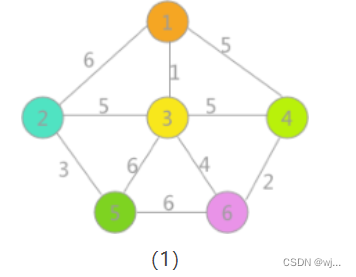6 10
1
2
3
4
5
6
1 2 6
1 3 1
1 4 5
2 3 5
2 5 3
3 4 5
3 5 6
3 6 4
4 6 2
5 6 6

1->3,1
4->6,2
2->5,3
3->6,4
2->3,5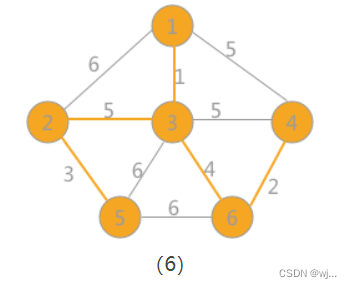06-27
11-043783
11-10741
03-2995
07-181930
04-021万+
12-22276
12-082622
06-114772
06-294882
09-261994
12-21
12-23
07-17791
03-15579
12-273万+
11-189万+

### “相关推荐”对你有帮助么？

•非常没帮助
•没帮助
•一般
•有帮助
•非常有帮助被折叠的  条评论 为什么被折叠?到【灌水乐园】发言wj...

¥2 ¥4 ¥6 ¥10 ¥20余额支付 (余额：-- )扫码支付获取中扫码支付点击重新获取扫码支付1.余额是钱包充值的虚拟货币，按照1:1的比例进行支付金额的抵扣。
2.余额无法直接购买下载，可以购买VIP、C币套餐、付费专栏及课程。余额充值